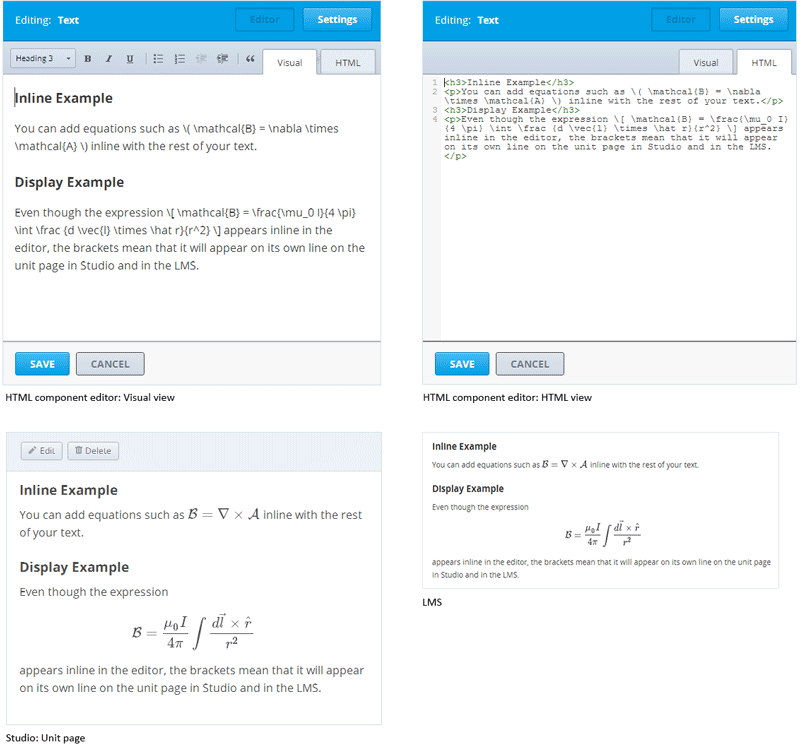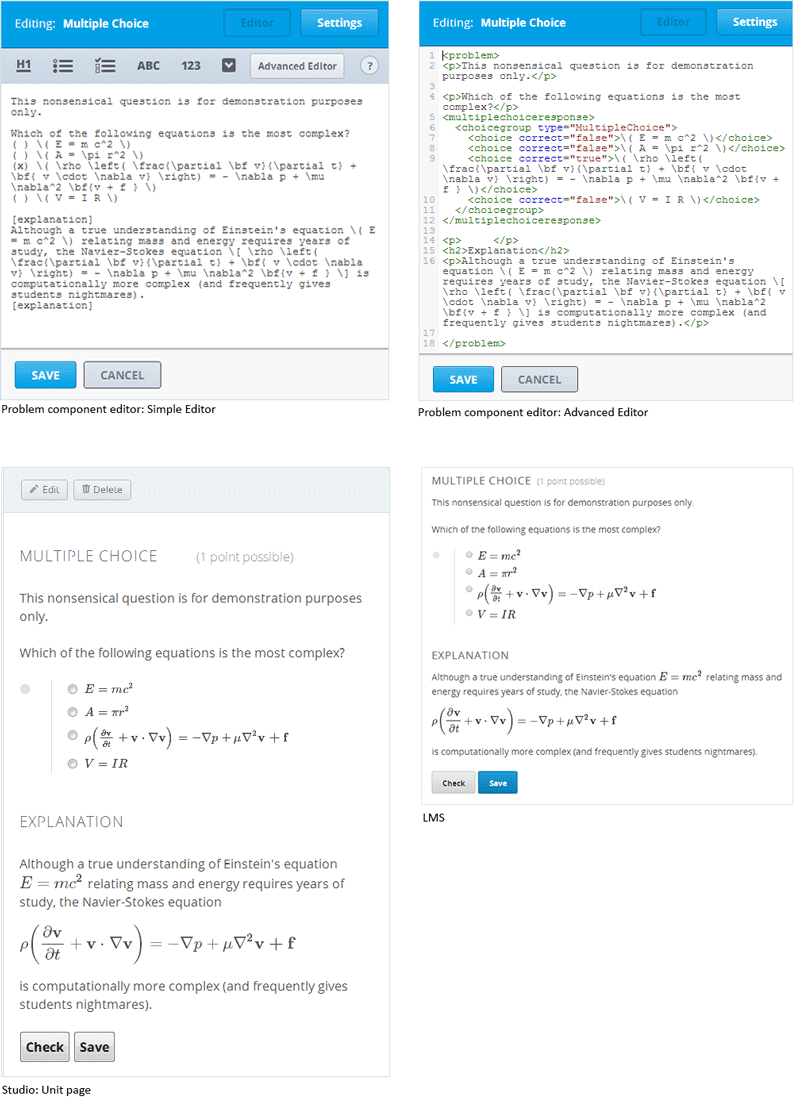# 7.41. A Brief Introduction to MathJax in Studio¶

To write clear and professional-looking symbols and equations, we use a LaTeX-like language called MathJax. Your MathJax equations can appear with other text in the paragraph (inline equations) or on their own lines (display equations).

• For inline equations, you can do either of the following.

• Surround your Mathjax expression with backslashes and parentheses.

$$equation$$

• Surround your Mathjax expression with [mathjaxinline] tags. Note that these tags use square brackets ([]).

[mathjaxinline] equation [/mathjaxinline]

• For display equations, you can do either of the following.

• Surround your Mathjax expression with backslashes and brackets.

$equation$

• Surround your Mathjax expression with [mathjax] tags. Note that these tags use square brackets ([]).

[mathjax] equation [/mathjax]

You can use MathJax in HTML (text) components and in Problem components.

Note

Complete MathJax documentation (together with a testing tool) can be found at http://www.onemathematicalcat.org/MathJaxDocumentation/TeXSyntax.htm.

## 7.41.1. HTML (Text) Components¶

In the HTML component editor, you can use MathJax both in Visual view and in HTML view.## 7.41.2. Problem Components¶

In the Problem component editor, you can use MathJax both in the Simple Editor and in the Advanced Editor.

In the example problem below, note that the Einstein equation in the explanation is enclosed in backslashes and parentheses, so it appears inline with the text. The Navier-Stokes equation is enclosed in backslashes and brackets, so it appears on its own line.